Скачать презентацию Contracting Under Imperfect Commitment Ph D 279 B

adfda0c0a351c568ce3bb0c9b33a2711.ppt

• Количество слайдов: 39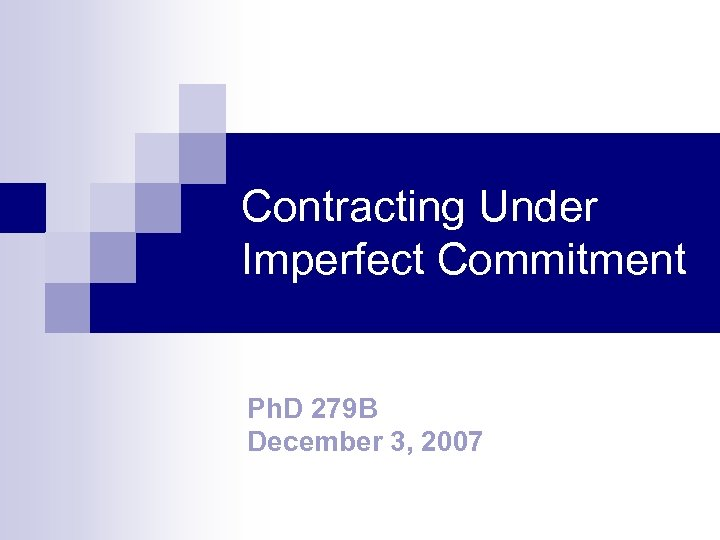Contracting Under Imperfect Commitment Ph. D 279 B December 3, 2007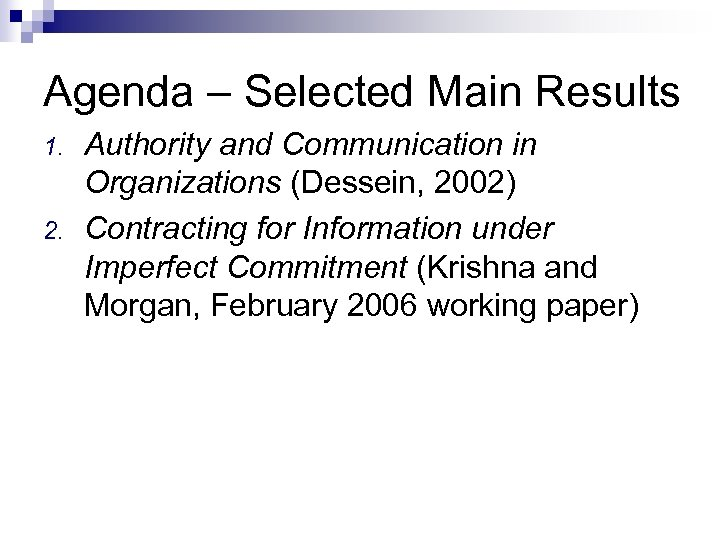Agenda – Selected Main Results 1. 2. Authority and Communication in Organizations (Dessein, 2002) Contracting for Information under Imperfect Commitment (Krishna and Morgan, February 2006 working paper)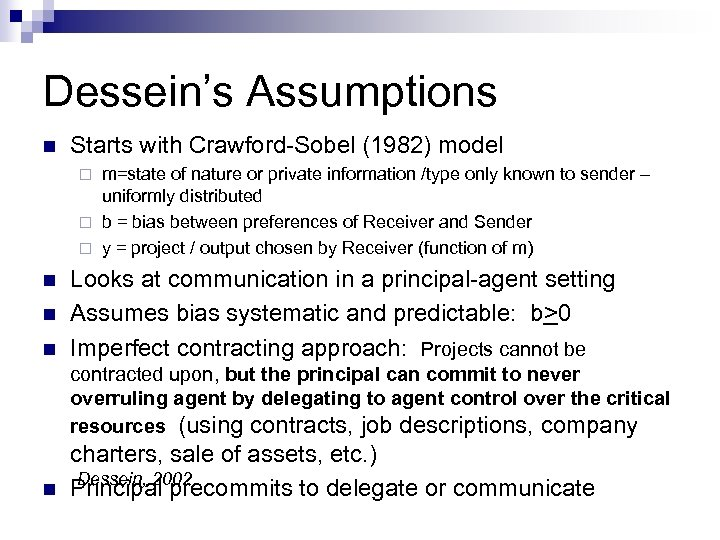Dessein’s Assumptions n Starts with Crawford-Sobel (1982) model m=state of nature or private information /type only known to sender – uniformly distributed ¨ b = bias between preferences of Receiver and Sender ¨ y = project / output chosen by Receiver (function of m) ¨ n n n Looks at communication in a principal-agent setting Assumes bias systematic and predictable: b>0 Imperfect contracting approach: Projects cannot be contracted upon, but the principal can commit to never overruling agent by delegating to agent control over the critical resources (using contracts, job descriptions, company n charters, sale of assets, etc. ) Dessein, 2002 Principal precommits to delegate or communicate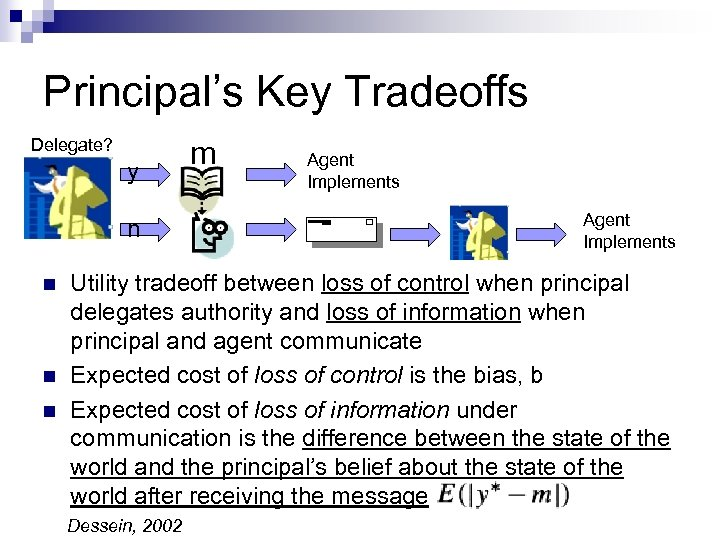Principal’s Key Tradeoffs Delegate? y n n m Agent Implements Utility tradeoff between loss of control when principal delegates authority and loss of information when principal and agent communicate Expected cost of loss of control is the bias, b Expected cost of loss of information under communication is the difference between the state of the world and the principal’s belief about the state of the world after receiving the message Dessein, 2002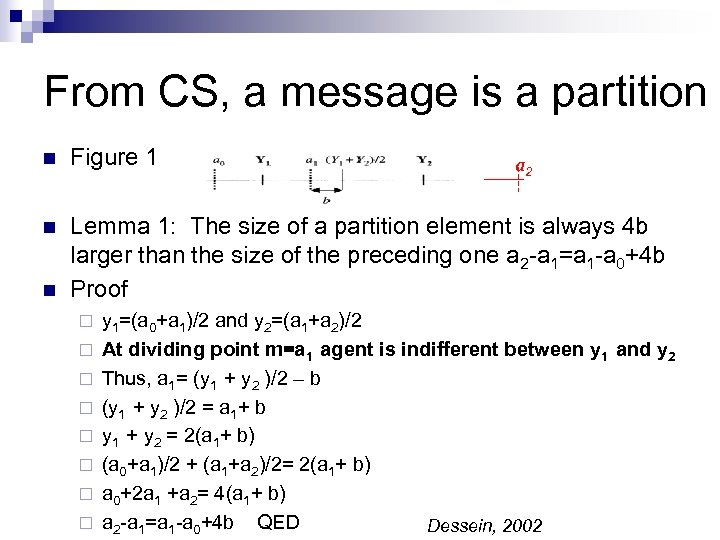From CS, a message is a partition n Figure 1 n Lemma 1: The size of a partition element is always 4 b larger than the size of the preceding one a 2 -a 1=a 1 -a 0+4 b Proof n ¨ ¨ ¨ ¨ a 2 y 1=(a 0+a 1)/2 and y 2=(a 1+a 2)/2 At dividing point m=a 1 agent is indifferent between y 1 and y 2 Thus, a 1= (y 1 + y 2 )/2 – b (y 1 + y 2 )/2 = a 1+ b y 1 + y 2 = 2(a 1+ b) (a 0+a 1)/2 + (a 1+a 2)/2= 2(a 1+ b) a 0+2 a 1 +a 2= 4(a 1+ b) a 2 -a 1=a 1 -a 0+4 b QED Dessein, 2002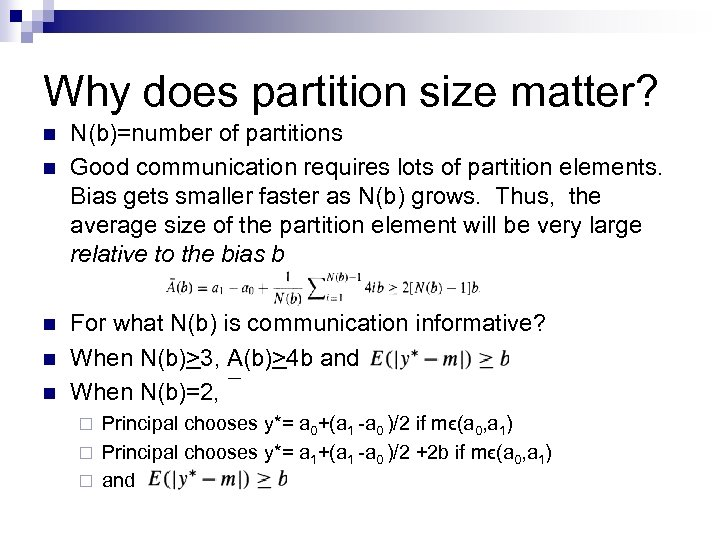Why does partition size matter? n n n N(b)=number of partitions Good communication requires lots of partition elements. Bias gets smaller faster as N(b) grows. Thus, the average size of the partition element will be very large relative to the bias b For what N(b) is communication informative? When N(b)>3, A(b)>4 b and When N(b)=2, Principal chooses y*= a 0+(a 1 -a 0 )/2 if mϵ(a 0, a 1) ¨ Principal chooses y*= a 1+(a 1 -a 0 )/2 +2 b if mϵ(a 0, a 1) ¨ and ¨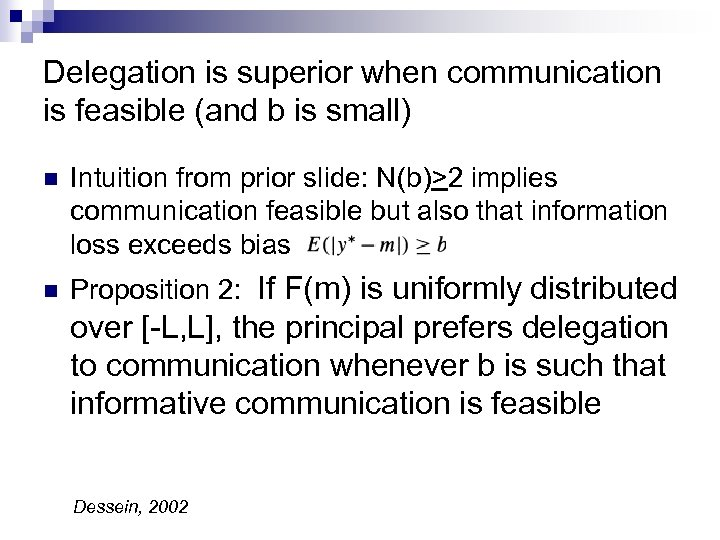Delegation is superior when communication is feasible (and b is small) n Intuition from prior slide: N(b)>2 implies communication feasible but also that information loss exceeds bias n Proposition 2: If F(m) is uniformly distributed over [-L, L], the principal prefers delegation to communication whenever b is such that informative communication is feasible Dessein, 2002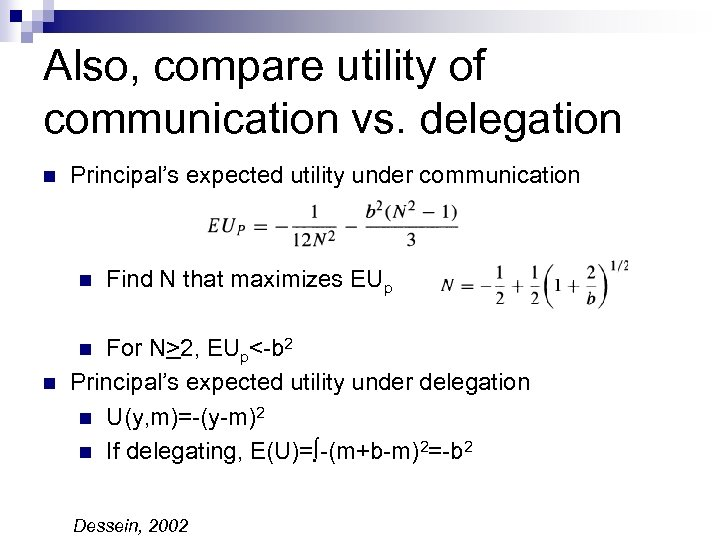Also, compare utility of communication vs. delegation n Principal’s expected utility under communication n Find N that maximizes EUp For N>2, EUp<-b 2 Principal’s expected utility under delegation n U(y, m)=-(y-m)2 n If delegating, E(U)=∫-(m+b-m)2=-b 2 n n Dessein, 2002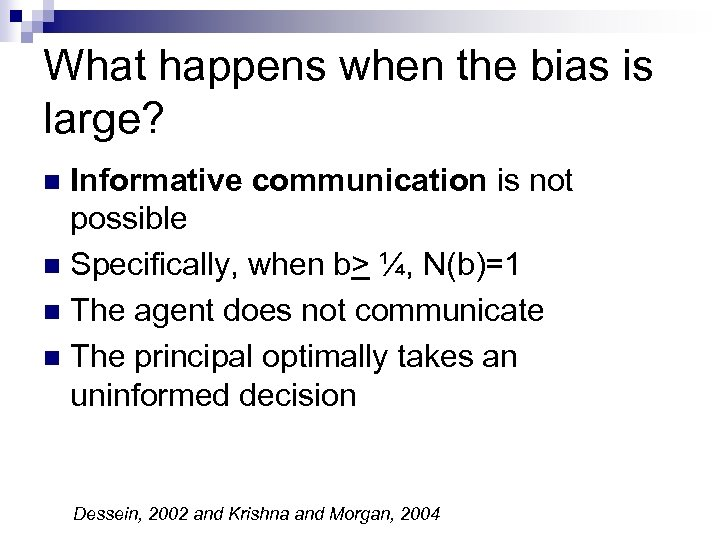What happens when the bias is large? Informative communication is not possible n Specifically, when b> ¼, N(b)=1 n The agent does not communicate n The principal optimally takes an uninformed decision n Dessein, 2002 and Krishna and Morgan, 2004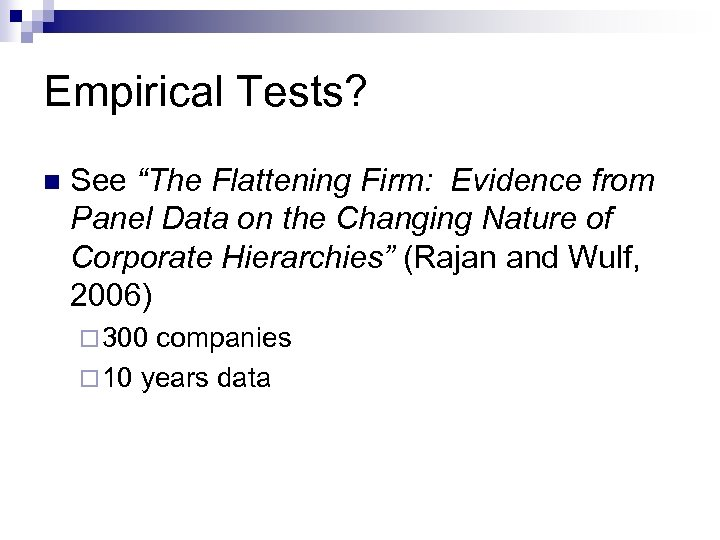Empirical Tests? n See “The Flattening Firm: Evidence from Panel Data on the Changing Nature of Corporate Hierarchies” (Rajan and Wulf, 2006) ¨ 300 companies ¨ 10 years data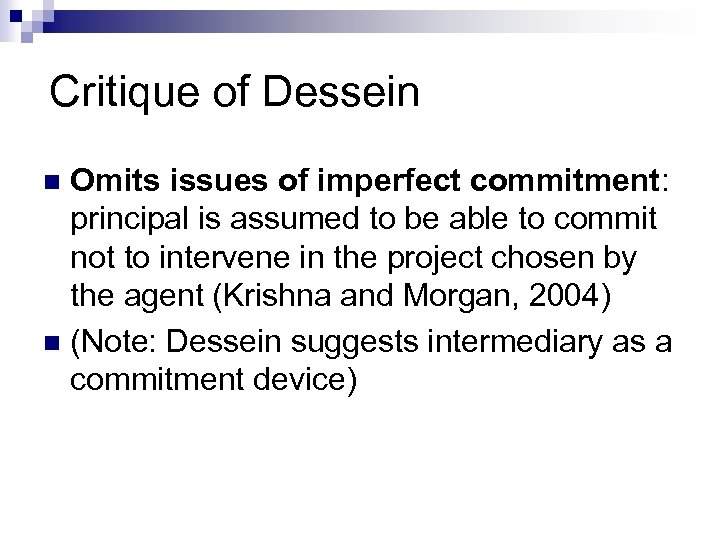Critique of Dessein Omits issues of imperfect commitment: principal is assumed to be able to commit not to intervene in the project chosen by the agent (Krishna and Morgan, 2004) n (Note: Dessein suggests intermediary as a commitment device) n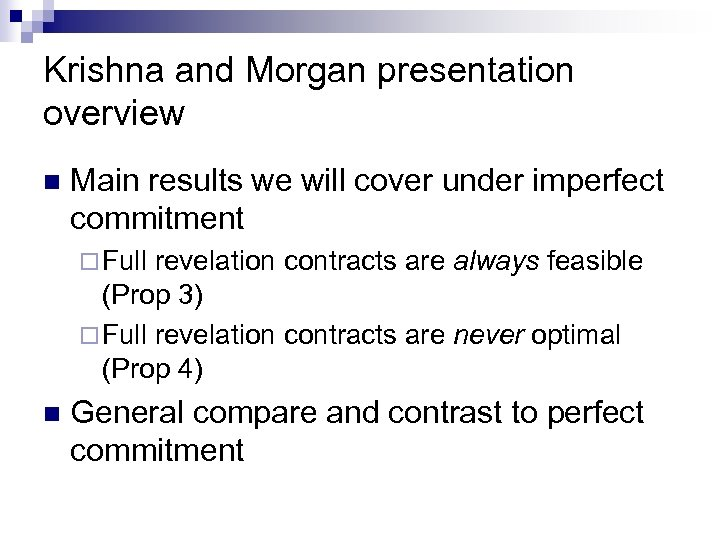Krishna and Morgan presentation overview n Main results we will cover under imperfect commitment ¨ Full revelation contracts are always feasible (Prop 3) ¨ Full revelation contracts are never optimal (Prop 4) n General compare and contrast to perfect commitment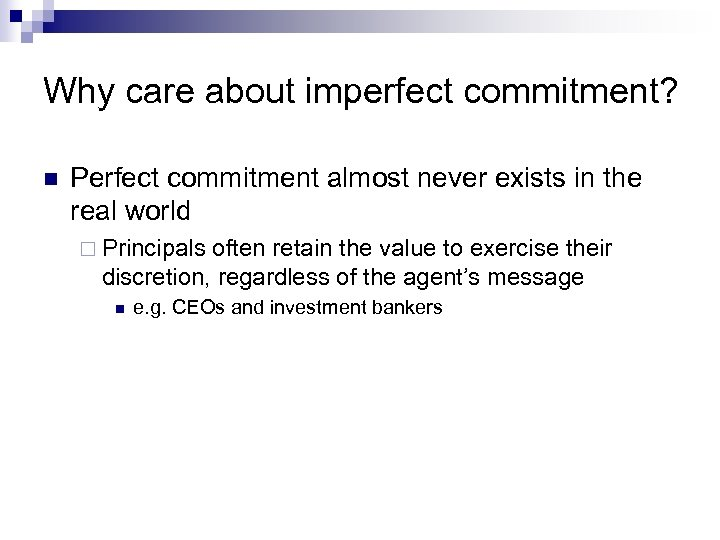Why care about imperfect commitment? n Perfect commitment almost never exists in the real world ¨ Principals often retain the value to exercise their discretion, regardless of the agent’s message n e. g. CEOs and investment bankersHow Krishna and Morgan explore imperfect commitment n n n Perfect commitment has been a critical assumption in mechanism design because it allows us to use the standard revelation principle However, under imperfect commitment, if agents reveal truthfully, principals are free to use the information to their own advantage. Knowing this, agents will generally be better off not revealing the whole truth In imperfect commitment, Krishna and Morgan assume the set of types Θ is a continuum, and derive a partial version of the revelation principle to get their results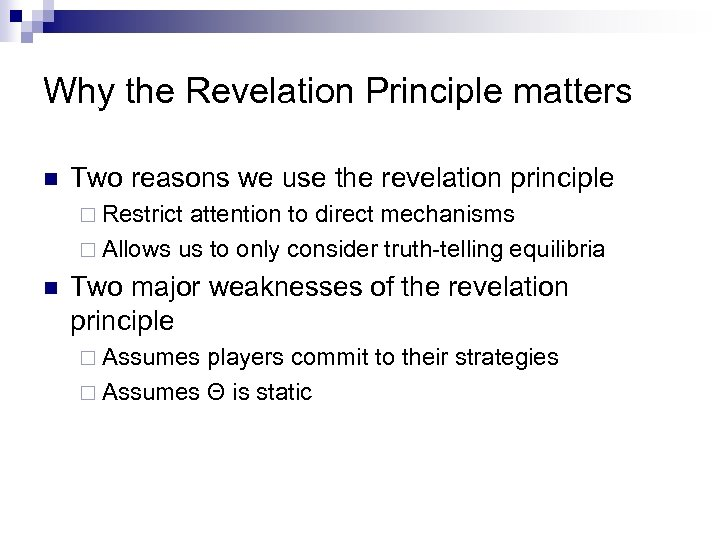Why the Revelation Principle matters n Two reasons we use the revelation principle ¨ Restrict attention to direct mechanisms ¨ Allows us to only consider truth-telling equilibria n Two major weaknesses of the revelation principle ¨ Assumes players commit to their strategies ¨ Assumes Θ is static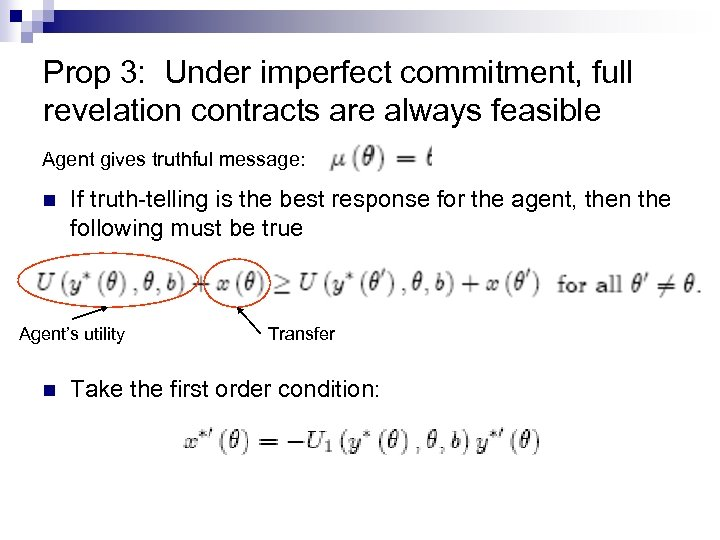Prop 3: Under imperfect commitment, full revelation contracts are always feasible Agent gives truthful message: n If truth-telling is the best response for the agent, then the following must be true Agent’s utility n Transfer Take the first order condition: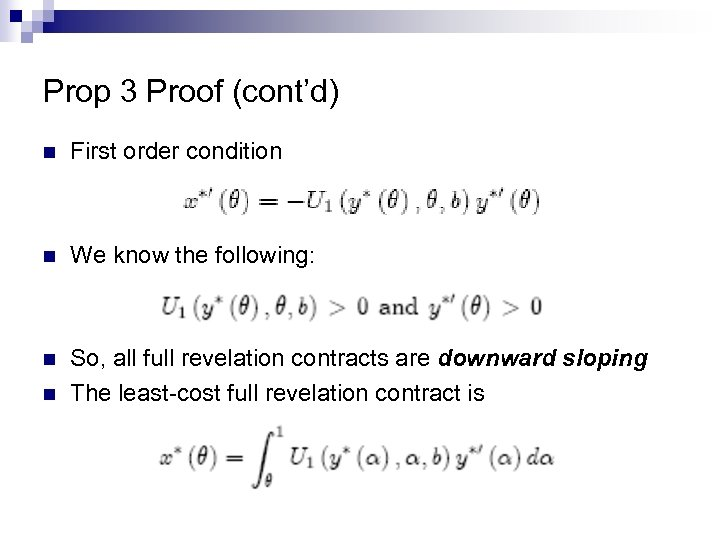Prop 3 Proof (cont’d) n First order condition n We know the following: n So, all full revelation contracts are downward sloping The least-cost full revelation contract is n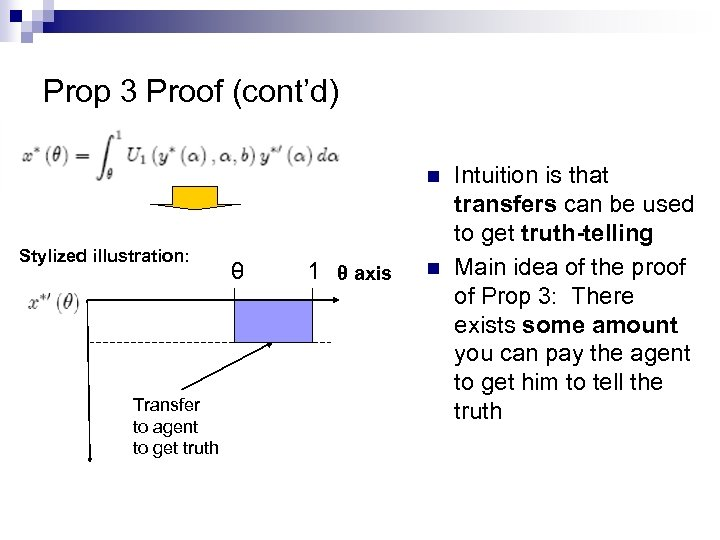Prop 3 Proof (cont’d) n Stylized illustration: Transfer to agent to get truth θ 1 θ axis n Intuition is that transfers can be used to get truth-telling Main idea of the proof of Prop 3: There exists some amount you can pay the agent to get him to tell the truth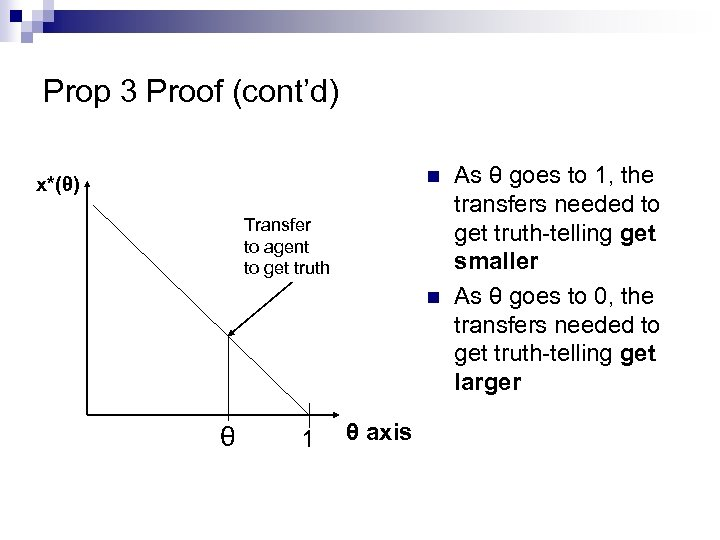Prop 3 Proof (cont’d) n x*(θ) Transfer to agent to get truth n θ 1 θ axis As θ goes to 1, the transfers needed to get truth-telling get smaller As θ goes to 0, the transfers needed to get truth-telling get larger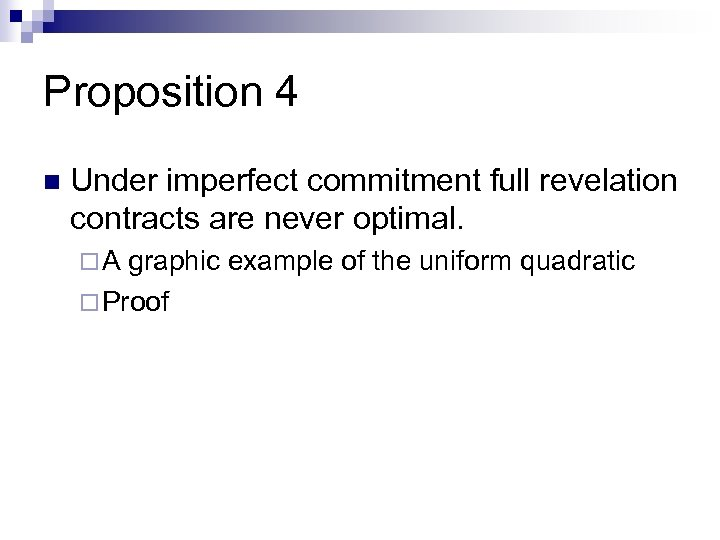Proposition 4 n Under imperfect commitment full revelation contracts are never optimal. ¨A graphic example of the uniform quadratic ¨ Proof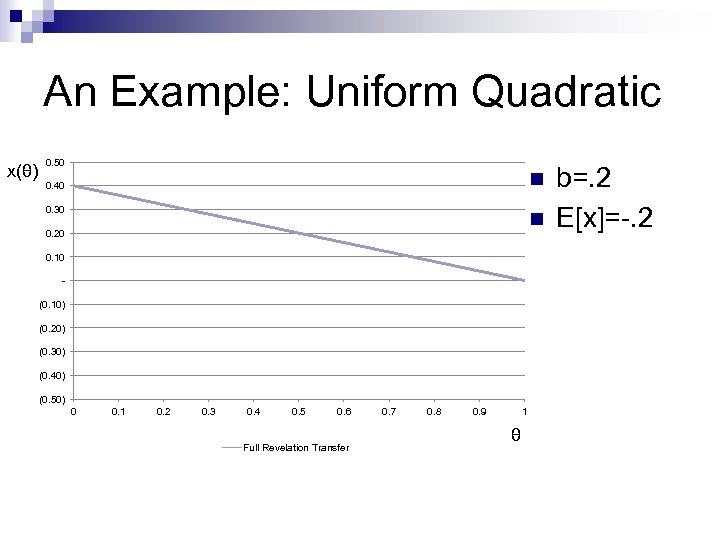An Example: Uniform Quadratic x(θ) 0. 50 n 0. 40 0. 30 n 0. 20 0. 10 (0. 10) (0. 20) (0. 30) (0. 40) (0. 50) 0 0. 1 0. 2 0. 3 0. 4 0. 5 0. 6 Full Revelation Transfer 0. 7 0. 8 0. 9 1 θ b=. 2 E[x]=-. 2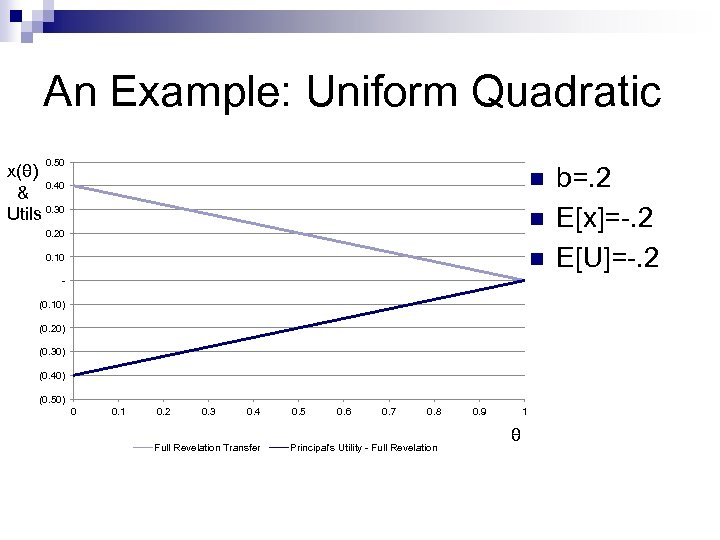An Example: Uniform Quadratic 0. 50 x(θ) 0. 40 & Utils 0. 30 n n 0. 20 n 0. 10 (0. 10) (0. 20) (0. 30) (0. 40) (0. 50) 0 0. 1 0. 2 0. 3 0. 4 Full Revelation Transfer 0. 5 0. 6 0. 7 0. 8 Principal's Utility - Full Revelation 0. 9 1 θ b=. 2 E[x]=-. 2 E[U]=-. 2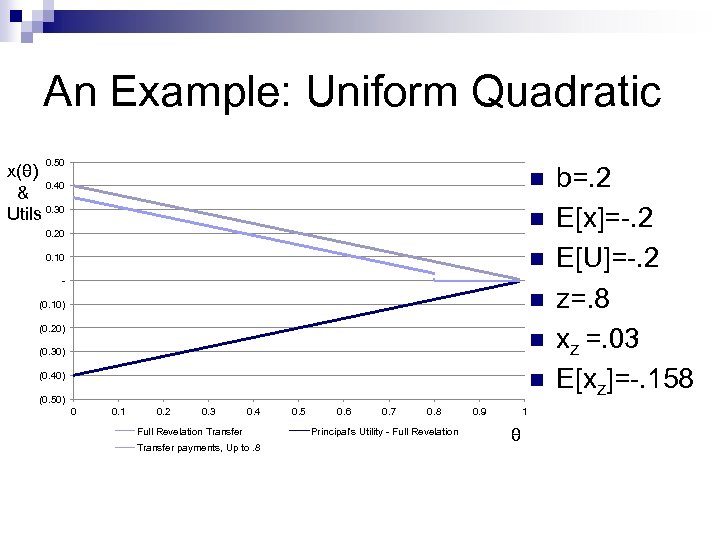An Example: Uniform Quadratic 0. 50 x(θ) 0. 40 & Utils 0. 30 n n 0. 20 n 0. 10 - n (0. 10) (0. 20) n (0. 30) n (0. 40) (0. 50) 0 0. 1 0. 2 0. 3 0. 4 Full Revelation Transfer payments, Up to. 8 0. 5 0. 6 0. 7 0. 8 Principal's Utility - Full Revelation 0. 9 1 θ b=. 2 E[x]=-. 2 E[U]=-. 2 z=. 8 xz =. 03 E[xz]=-. 158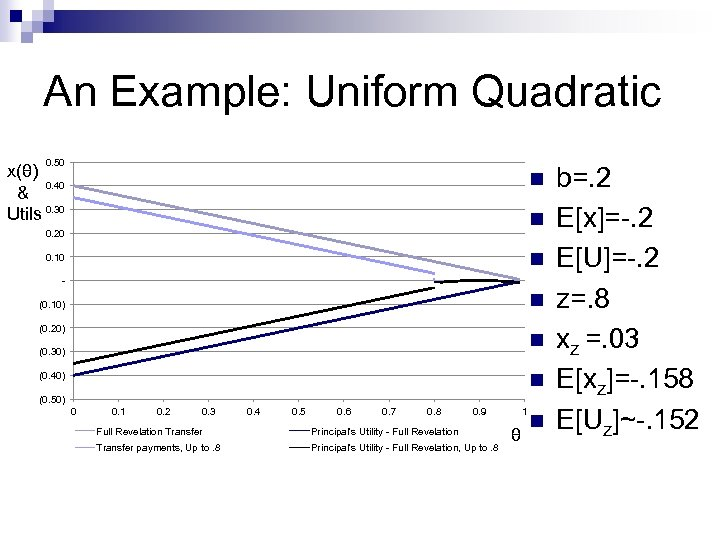An Example: Uniform Quadratic 0. 50 x(θ) 0. 40 & Utils 0. 30 n n 0. 20 n 0. 10 - n (0. 10) (0. 20) n (0. 30) n (0. 40) (0. 50) 0 0. 1 0. 2 0. 3 0. 4 0. 5 0. 6 0. 7 0. 8 0. 9 Full Revelation Transfer Principal's Utility - Full Revelation Transfer payments, Up to. 8 Principal's Utility - Full Revelation, Up to. 8 1 θ n b=. 2 E[x]=-. 2 E[U]=-. 2 z=. 8 xz =. 03 E[xz]=-. 158 E[Uz]~-. 152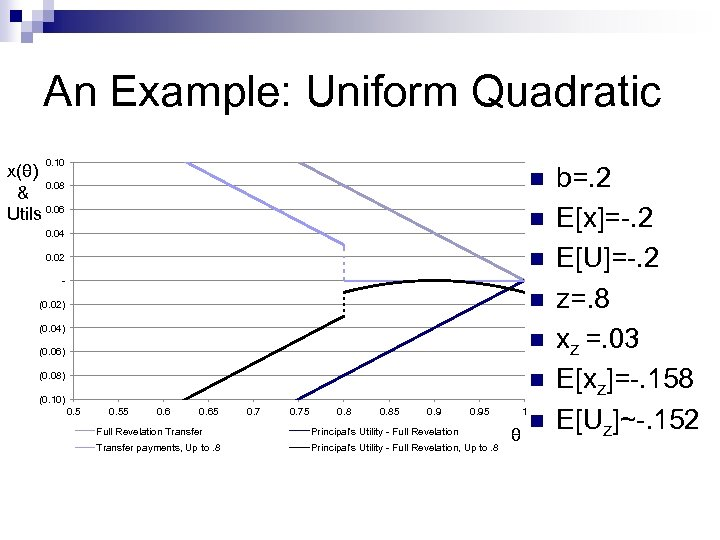An Example: Uniform Quadratic 0. 10 x(θ) 0. 08 & Utils 0. 06 n n 0. 04 n 0. 02 - n (0. 02) (0. 04) n (0. 06) n (0. 08) (0. 10) 0. 55 0. 65 0. 75 0. 85 0. 95 Full Revelation Transfer Principal's Utility - Full Revelation Transfer payments, Up to. 8 Principal's Utility - Full Revelation, Up to. 8 1 θ n b=. 2 E[x]=-. 2 E[U]=-. 2 z=. 8 xz =. 03 E[xz]=-. 158 E[Uz]~-. 152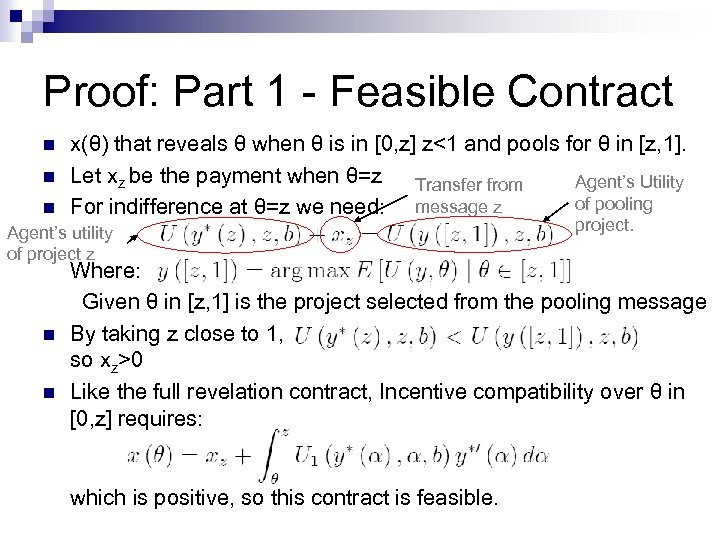Proof: Part 1 - Feasible Contract n n n x(θ) that reveals θ when θ is in [0, z] z<1 and pools for θ in [z, 1]. Let xz be the payment when θ=z Transfer from Agent’s Utility of pooling For indifference at θ=z we need: message z Agent’s utility of project z n n project. Where: Given θ in [z, 1] is the project selected from the pooling message By taking z close to 1, so xz>0 Like the full revelation contract, Incentive compatibility over θ in [0, z] requires: which is positive, so this contract is feasible.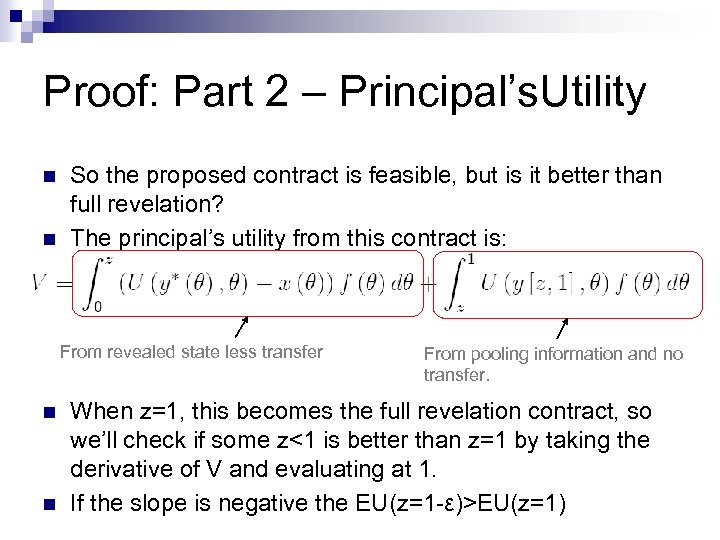Proof: Part 2 – Principal’s. Utility n n So the proposed contract is feasible, but is it better than full revelation? The principal’s utility from this contract is: From revealed state less transfer n n From pooling information and no transfer. When z=1, this becomes the full revelation contract, so we’ll check if some z<1 is better than z=1 by taking the derivative of V and evaluating at 1. If the slope is negative the EU(z=1 -ε)>EU(z=1)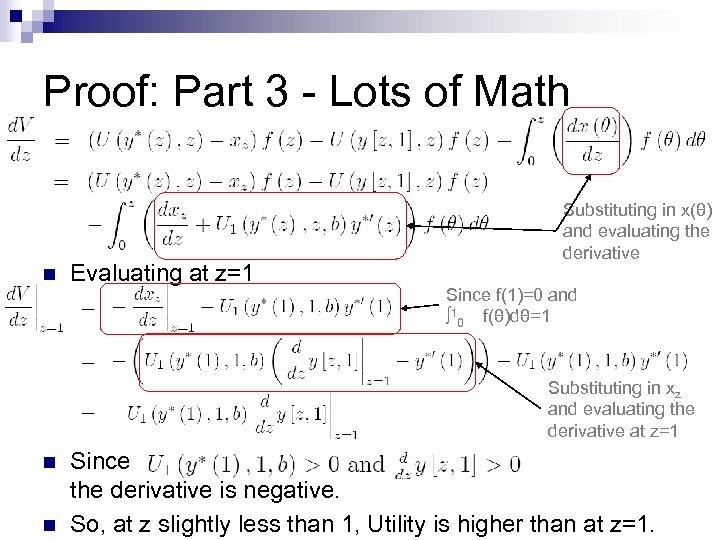Proof: Part 3 - Lots of Math n Evaluating at z=1 Substituting in x(θ) and evaluating the derivative Since f(1)=0 and ∫ 10 _f(θ)dθ=1 Substituting in xz and evaluating the derivative at z=1 n n Since the derivative is negative. So, at z slightly less than 1, Utility is higher than at z=1.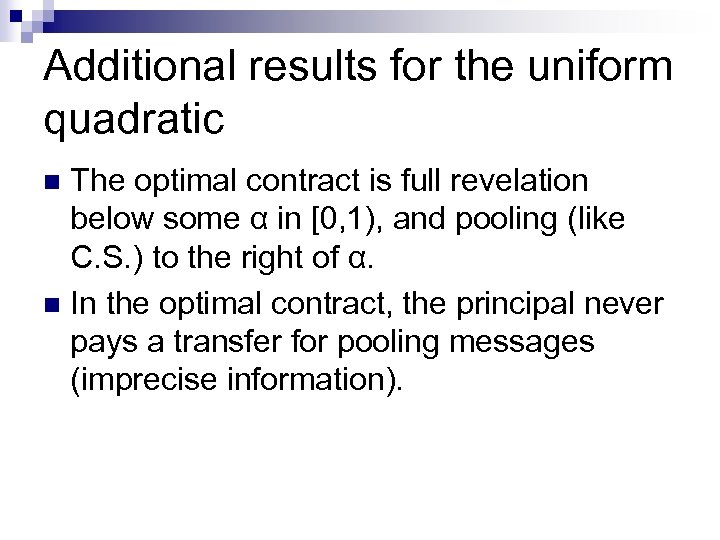Additional results for the uniform quadratic The optimal contract is full revelation below some α in [0, 1), and pooling (like C. S. ) to the right of α. n In the optimal contract, the principal never pays a transfer for pooling messages (imprecise information). n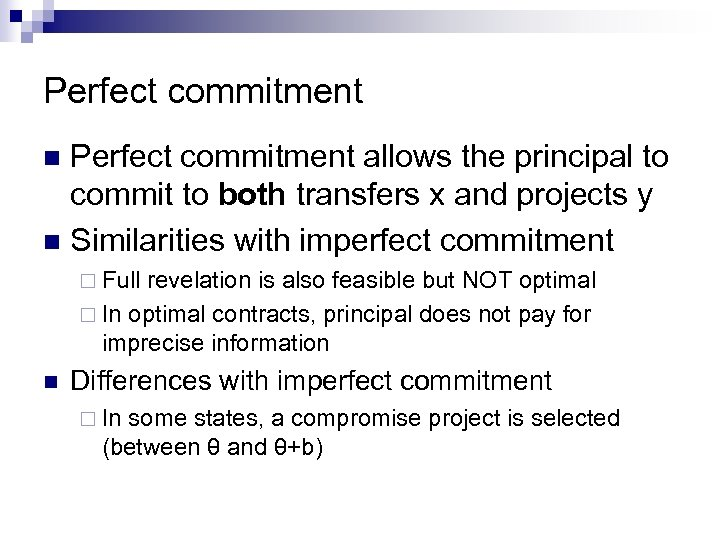Perfect commitment allows the principal to commit to both transfers x and projects y n Similarities with imperfect commitment n ¨ Full revelation is also feasible but NOT optimal ¨ In optimal contracts, principal does not pay for imprecise information n Differences with imperfect commitment ¨ In some states, a compromise project is selected (between θ and θ+b)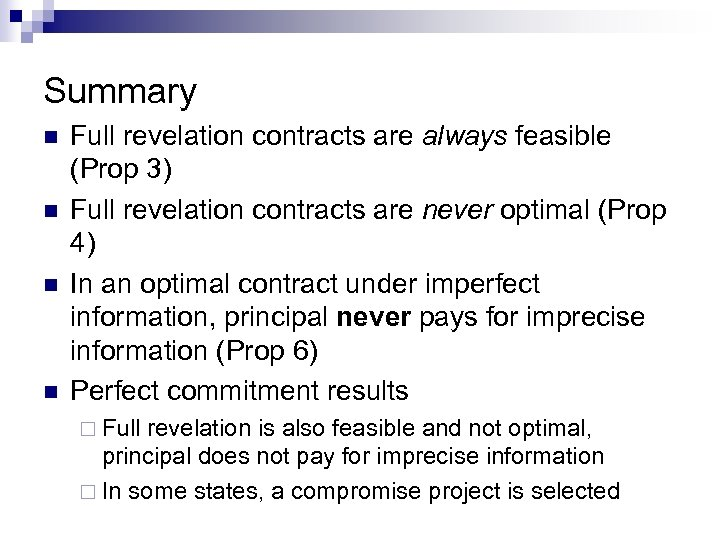Summary n n Full revelation contracts are always feasible (Prop 3) Full revelation contracts are never optimal (Prop 4) In an optimal contract under imperfect information, principal never pays for imprecise information (Prop 6) Perfect commitment results ¨ Full revelation is also feasible and not optimal, principal does not pay for imprecise information ¨ In some states, a compromise project is selectedAppendix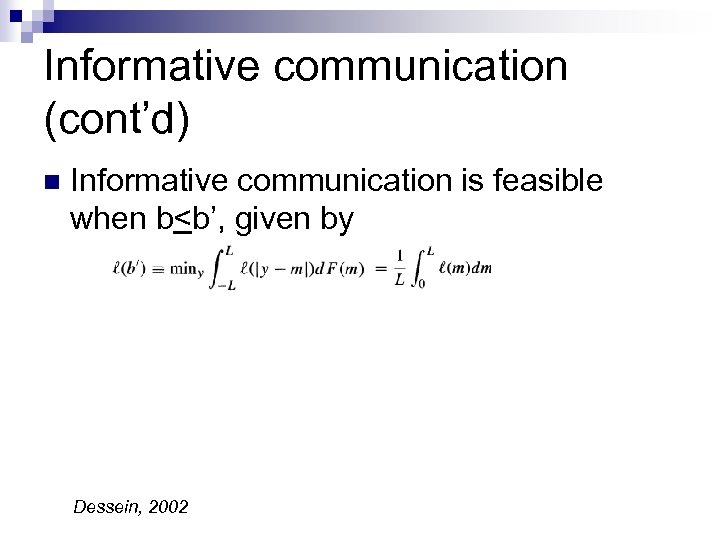Informative communication (cont’d) n Informative communication is feasible when b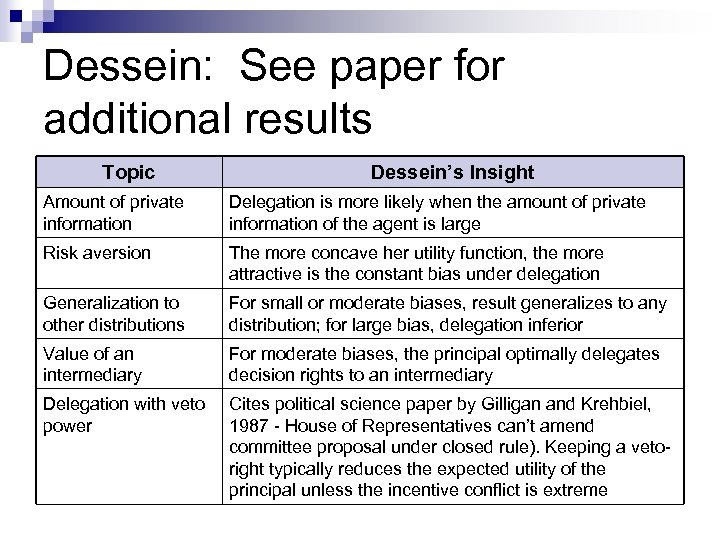Dessein: See paper for additional results Topic Dessein’s Insight Amount of private information Delegation is more likely when the amount of private information of the agent is large Risk aversion The more concave her utility function, the more attractive is the constant bias under delegation Generalization to other distributions For small or moderate biases, result generalizes to any distribution; for large bias, delegation inferior Value of an intermediary For moderate biases, the principal optimally delegates decision rights to an intermediary Delegation with veto power Cites political science paper by Gilligan and Krehbiel, 1987 - House of Representatives can’t amend committee proposal under closed rule). Keeping a vetoright typically reduces the expected utility of the principal unless the incentive conflict is extreme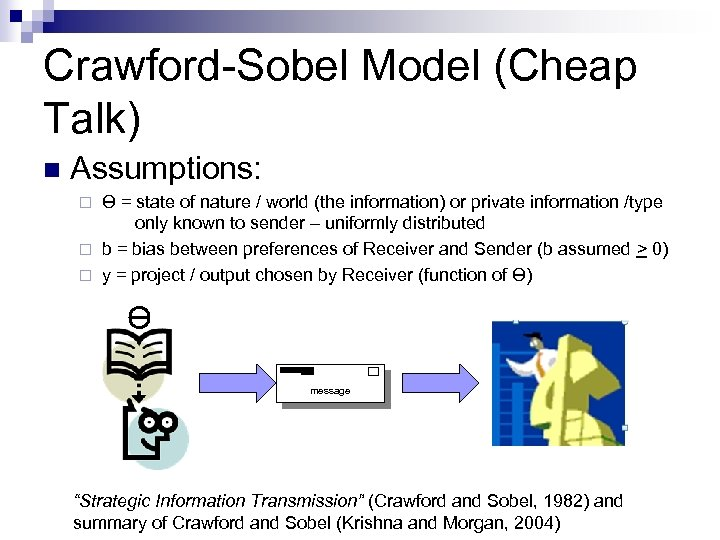Crawford-Sobel Model (Cheap Talk) n Assumptions: ϴ = state of nature / world (the information) or private information /type only known to sender – uniformly distributed ¨ b = bias between preferences of Receiver and Sender (b assumed > 0) ¨ y = project / output chosen by Receiver (function of ϴ) ¨ ϴ message “Strategic Information Transmission” (Crawford and Sobel, 1982) and summary of Crawford and Sobel (Krishna and Morgan, 2004)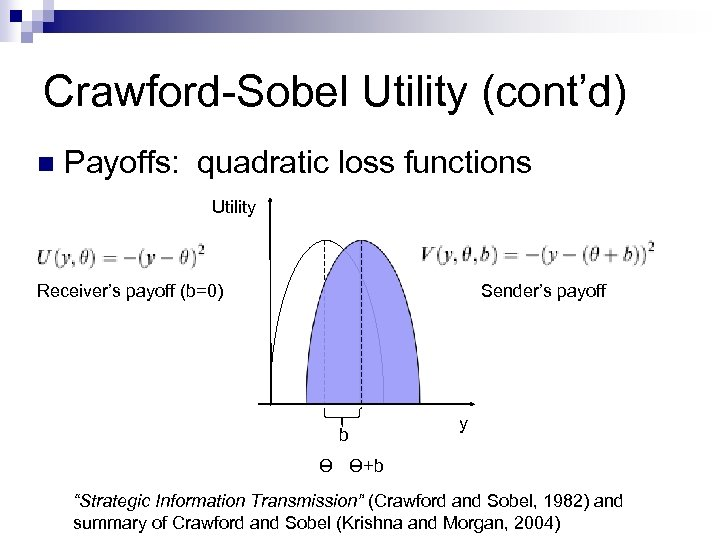Crawford-Sobel Utility (cont’d) n Payoffs: quadratic loss functions Utility Receiver’s payoff (b=0) Sender’s payoff b y ϴ ϴ+b “Strategic Information Transmission” (Crawford and Sobel, 1982) and summary of Crawford and Sobel (Krishna and Morgan, 2004)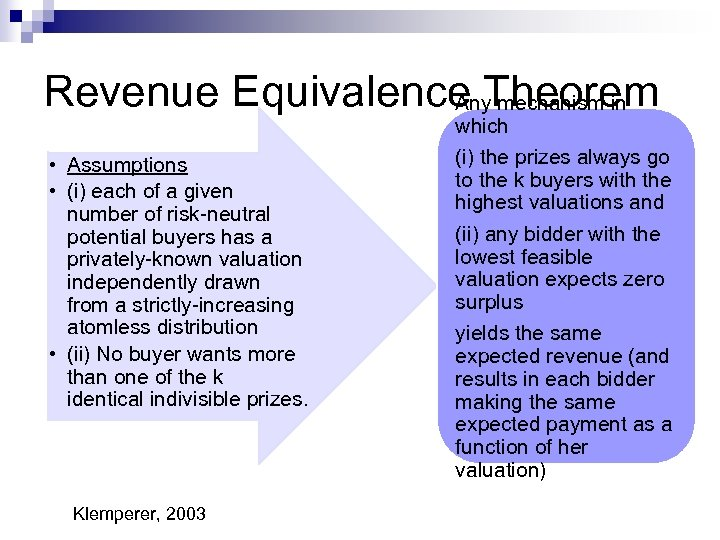Revenue Equivalence Theorem Any mechanism in • Assumptions • (i) each of a given number of risk-neutral potential buyers has a privately-known valuation independently drawn from a strictly-increasing atomless distribution • (ii) No buyer wants more than one of the k identical indivisible prizes. Klemperer, 2003 which (i) the prizes always go to the k buyers with the highest valuations and (ii) any bidder with the lowest feasible valuation expects zero surplus yields the same expected revenue (and results in each bidder making the same expected payment as a function of her valuation)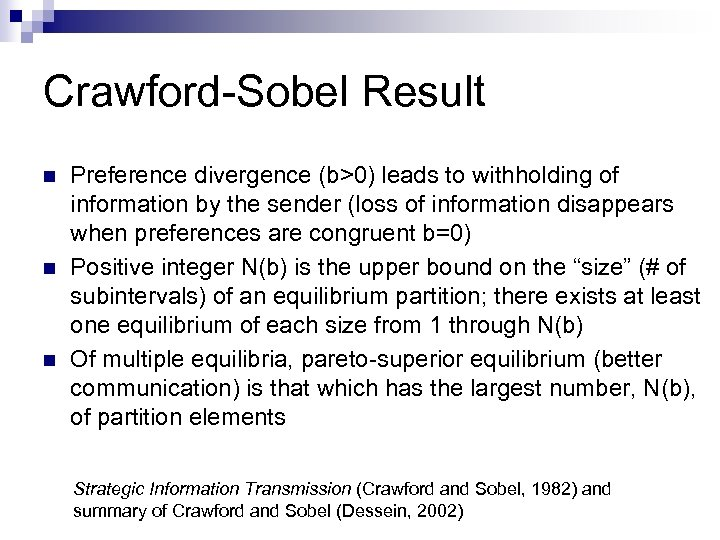Crawford-Sobel Result n n n Preference divergence (b>0) leads to withholding of information by the sender (loss of information disappears when preferences are congruent b=0) Positive integer N(b) is the upper bound on the “size” (# of subintervals) of an equilibrium partition; there exists at least one equilibrium of each size from 1 through N(b) Of multiple equilibria, pareto-superior equilibrium (better communication) is that which has the largest number, N(b), of partition elements Strategic Information Transmission (Crawford and Sobel, 1982) and summary of Crawford and Sobel (Dessein, 2002)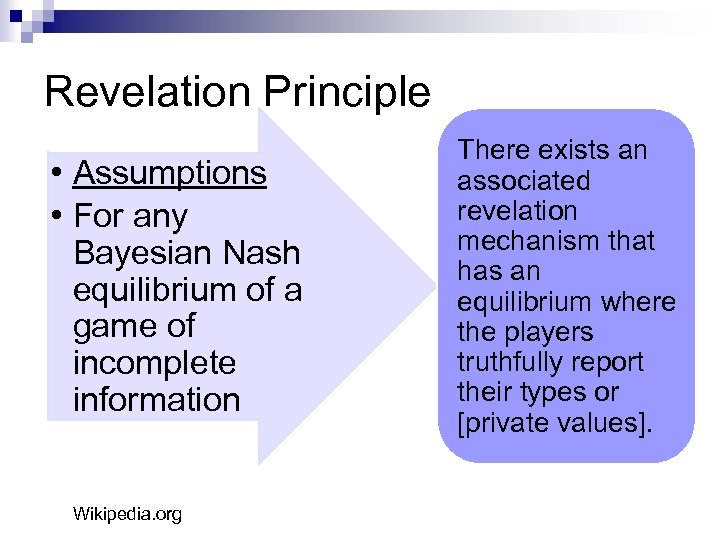Revelation Principle • Assumptions • For any Bayesian Nash equilibrium of a game of incomplete information Wikipedia. org There exists an associated revelation mechanism that has an equilibrium where the players truthfully report their types or [private values].